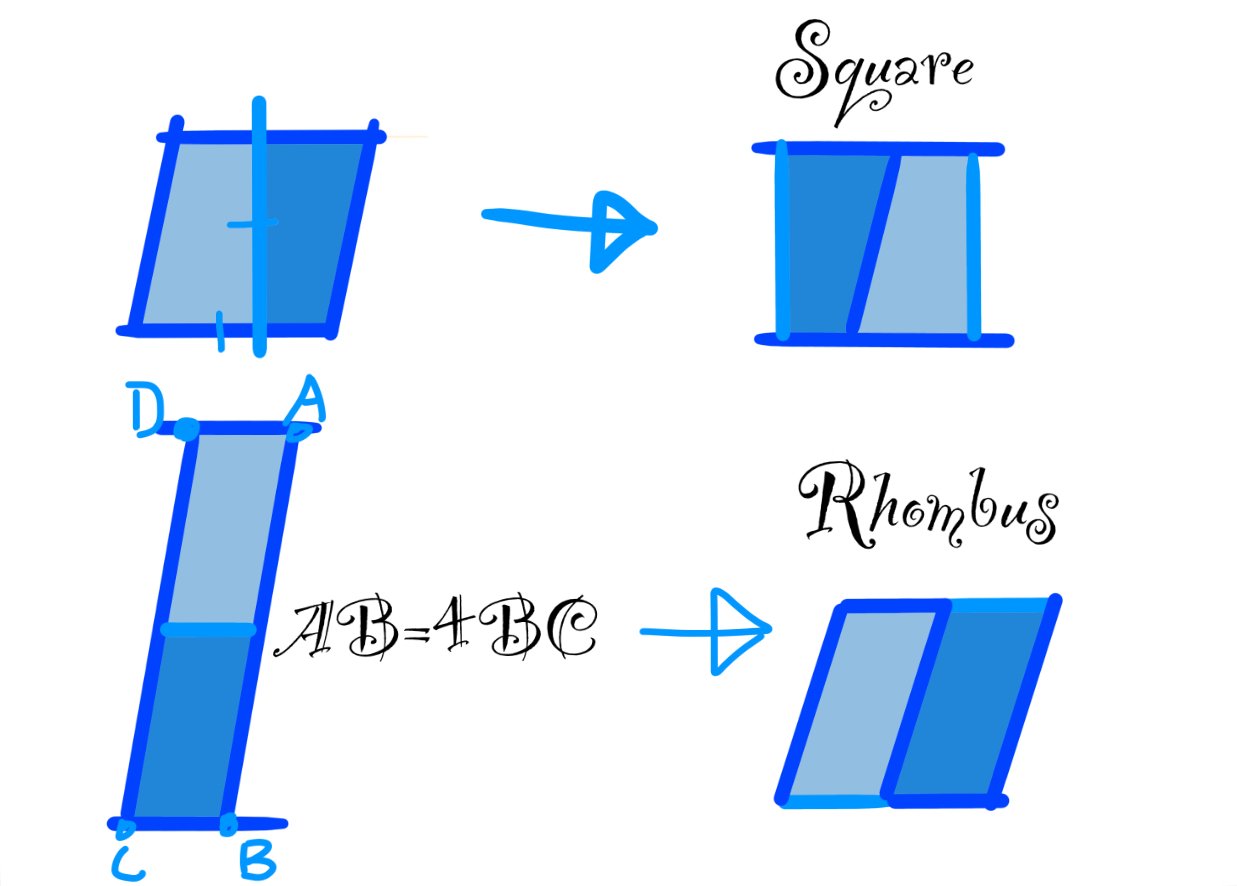I’ve come across a geometry problem which I think doesn’t have an answer. Am I wrong?
Here’s the problem:
Which of the following shapes cannot be obtained by cutting a parallelogram into two congruent pieces and rearranging into one?
A. Square (eliminated:base=height)
B. Rectangle (eliminated)
C. Rhombus (Xtra tricky but still eliminated:side AB=4$\times$side BC)
D. Parallelogram (eliminated)Note by Jeff Giff
1 year, 1 month ago

This discussion board is a place to discuss our Daily Challenges and the math and science related to those challenges. Explanations are more than just a solution — they should explain the steps and thinking strategies that you used to obtain the solution. Comments should further the discussion of math and science.

When posting on Brilliant:

• Use the emojis to react to an explanation, whether you're congratulating a job well done , or just really confused .
• Ask specific questions about the challenge or the steps in somebody's explanation. Well-posed questions can add a lot to the discussion, but posting "I don't understand!" doesn't help anyone.
• Try to contribute something new to the discussion, whether it is an extension, generalization or other idea related to the challenge.

MarkdownAppears as
*italics* or _italics_ italics
**bold** or __bold__ bold
- bulleted- list
• bulleted
• list
1. numbered2. list
1. numbered
2. list
Note: you must add a full line of space before and after lists for them to show up correctly
paragraph 1paragraph 2

paragraph 1

paragraph 2

[example link](https://brilliant.org)example link
> This is a quote
This is a quote
    # I indented these lines
# 4 spaces, and now they show
# up as a code block.

print "hello world"
# I indented these lines
# 4 spaces, and now they show
# up as a code block.

print "hello world"
MathAppears as
Remember to wrap math in $$ ... $$ or $ ... $ to ensure proper formatting.
2 \times 3 $2 \times 3$
2^{34} $2^{34}$
a_{i-1} $a_{i-1}$
\frac{2}{3} $\frac{2}{3}$
\sqrt{2} $\sqrt{2}$
\sum_{i=1}^3 $\sum_{i=1}^3$
\sin \theta $\sin \theta$
\boxed{123} $\boxed{123}$

Sort by:

Your solution definitely seems viable, maybe they meant that one shape can't be formed from any arbitrary parallelogram? In your examples you relied on certain proportions in the shape, so it might be the case that a construction doesn't exist for all parallelograms to turn into one of those shapes.

I think you can still eliminate rectangle and parallelogram though, because there can only be one answer and if either B or D were impossible, A or C would have to be as well.

- 1 year, 1 month ago